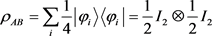量子纠缠的相关性判据The Correlation Criterion of QuantumEntanglement

• 全文下载: PDF(393KB)    PP.19-22   DOI: 10.12677/MP.2019.91003
• 下载量: 217  浏览量: 478

The separability judgment of quantum states is the basic problem of quantum entanglement theory. In this paper, based on Schmidt decomposition of two-body pure states, the correlation criteria of matrix rank and vector group are given. For the two-body mixed state, a sufficient and unnecessary condition for separability is given, and an example is given.

1. 引言

2. 量子纯态与复合系统

${|\phi 〉}_{AB}=\underset{i}{\sum }\underset{j}{\sum }{m}_{ij}|i〉〈j|$

3. 量子纯态纠缠

$\rho =|\phi 〉〈\phi |=\left({\xi }_{1},{\xi }_{2},\cdots ,{\xi }_{n}\right)$

4. 量子混合态纠缠

$\rho =\underset{i}{\sum }{p}_{i}|{\phi }_{i}〉〈{\phi }_{i}|$Michael, A., Chuang, N.I.L., 赵千川. 量子计算与量子信息[M]. 北京: 清华大学出版社, 2004.  Johnston, N., Kribs, D.W. and Teng, C.W. (2009) Operator Algebraic Formulation of the Stabilizer Formalism for Quantum Error Correction. Acta Applicandae Mathematicae, 108, 687-696. https://doi.org/10.1007/s10440-008-9421-1  Kribs, D.W., Laflamme, R., Poulin, D. and Lesosky, M. (2006) Operator Quantum Error Correction. Quantum Information and Computation, 6, 382-399.  张成杰. 量子纠缠的判定与度量[D]: [博士学位论文]. 合肥: 中国科学技术大学, 2010.  郑玉鳞. 量子纠缠与量子导引的判据研究[D]: [博士学位论文]. 合肥: 中国科学技术大学, 2016.